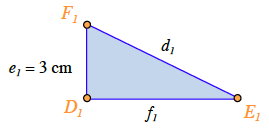### Home > PC > Chapter 2 > Lesson 2.1.2 > Problem2-29

2-29.
1. Solve each triangle. Homework Help ✎

1. Find the legs of an isosceles right triangle with a 10 cm hypotenuse.

2. Find the short leg of a 30°­60°­90° triangle with an 8 cm hypotenuse.

3. Find the long leg of a 30°­60°­90° triangle with a 3 cm short leg.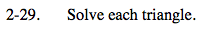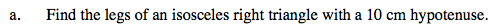Draw and label a sketch

Since you know the two legs are equal, what famous theorem starting with a 'p' can be useful in setting up an equation and solving?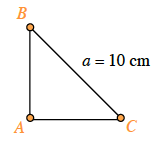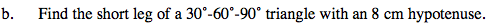Draw and label the triangle.
Which side is the short leg?

Set up a trig function with the known information and leg e. Solve for e.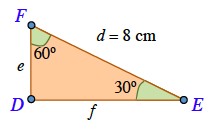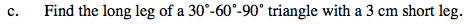f1 = 3√3 cm